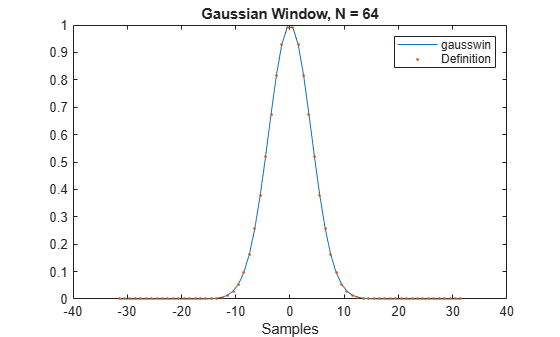# gausswin

Gaussian window

## Syntax

w = gausswin(L)
w = gausswin(L,alpha)

## Description

example

w = gausswin(L) returns an L-point Gaussian window.
w = gausswin(L,alpha) returns an L-point Gaussian window with width factor alpha. NoteIf the window appears to be clipped, increase L, the number of points. 

## Examples

collapse all

Create a 64-point Gaussian window. Display the result in wvtool.

L = 64; wvtool(gausswin(L))This example shows that the Fourier transform of the Gaussian window is also Gaussian with a reciprocal standard deviation. This is an illustration of the time-frequency uncertainty principle.

Create a Gaussian window of length $\mathit{N}=64$ by using gausswin and the defining equation. Set $\alpha =8$, which results in a standard deviation of $\left(\mathit{N}-1\right)/2\alpha =63/16$. The Gaussian is essentially limited to the mean plus or minus 3 standard deviations, or an approximate support of [–12, 12].

N = 64; n = -(N-1)/2:(N-1)/2; alpha = 8; w = gausswin(N,alpha); stdev = (N-1)/(2*alpha); y = exp(-1/2*(n/stdev).^2); plot(n,w) hold on plot(n,y,".") hold off xlabel("Samples") title("Gaussian Window, N = "+N) legend(["gausswin" "Definition"])Obtain the Fourier transform of the Gaussian window at $4\mathit{N}=256$ points. Use fftshift to center the Fourier transform at zero frequency (DC).

nfft = 4*N; freq = -pi:2*pi/nfft:pi-pi/nfft; wdft = fftshift(fft(w,nfft));

The Fourier transform of the Gaussian window is also Gaussian with a standard deviation that is the reciprocal of the time-domain standard deviation. Include the Gaussian normalization factor in your computation.

ydft = exp(-1/2*(freq/(1/stdev)).^2)*(stdev*sqrt(2*pi)); plot(freq/pi,abs(wdft)) hold on plot(freq/pi,abs(ydft),".") hold off xlabel("Normalized frequency (\times\pi rad/sample)") title("Fourier Transform of Gaussian Window") legend(["fft" "Definition"])## Input Arguments

collapse all

Window length, specified as a positive integer.

Data Types: single | double

Width factor, specified as a positive real scalar. alpha is inversely proportional to the width of the window.

Data Types: single | double

## Output Arguments

collapse all

Gaussian window, returned as a column vector.

## Algorithms

The coefficients of a Gaussian window are computed from the following equation:

$w\left(n\right)={e}^{-\frac{1}{2}{\left(\alpha \frac{n}{\left(L-1\right)/2}\right)}^{2}}={e}^{-{n}^{2}/2{\sigma }^{2}},$

where –(L – 1)/2 ≤ n ≤ (L – 1)/2, and α is inversely proportional to the standard deviation, σ, of a Gaussian random variable. The exact correspondence with the standard deviation of a Gaussian probability density function is σ = (L – 1)/(2α).

 Hansen, Eric W. Fourier Transforms: Principles and Applications. New York: John Wiley & Sons, 2014.

 Oppenheim, Alan V., Ronald W. Schafer, and John R. Buck. Discrete-Time Signal Processing. Upper Saddle River, NJ: Prentice Hall, 1999.